## Analyzing Patient Data

### Figure 9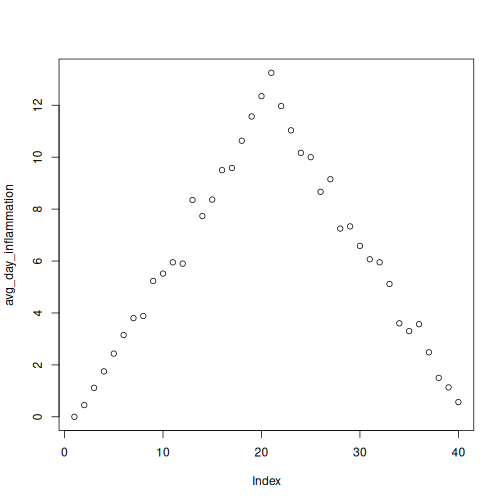Scatter plot of average inflammation versus time demonstrating the result of using the plot function

### Figure 10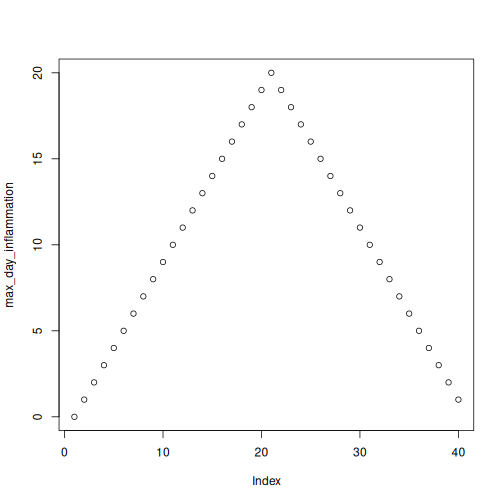Scatter plot of maximum inflammation versus time demonstrating the result of using the plot function

### Figure 11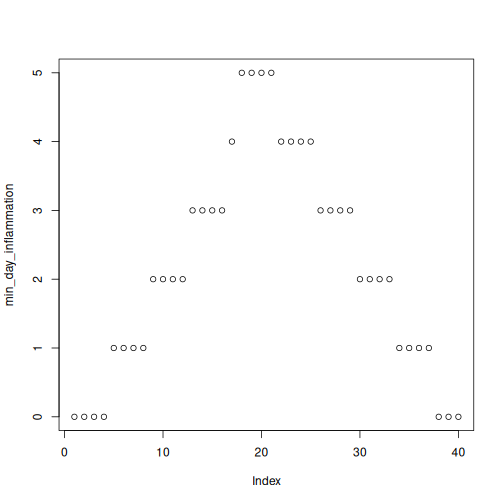Scatter plot of minimum inflammation versus time demonstrating the result of using the plot function

## Analyzing Multiple Data Sets

### Figure 1### Figure 2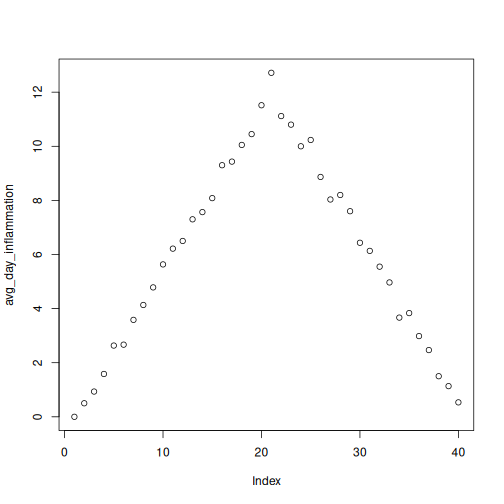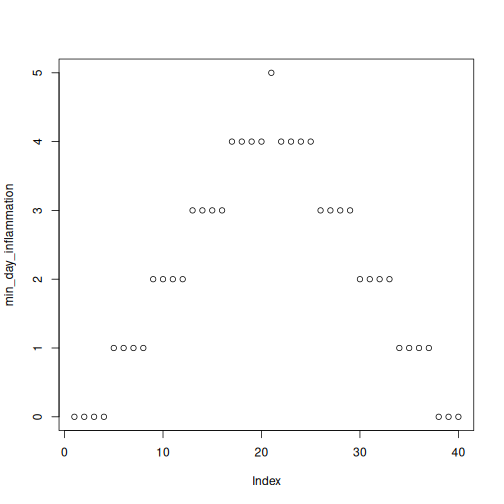### Figure 3### Figure 4### Figure 5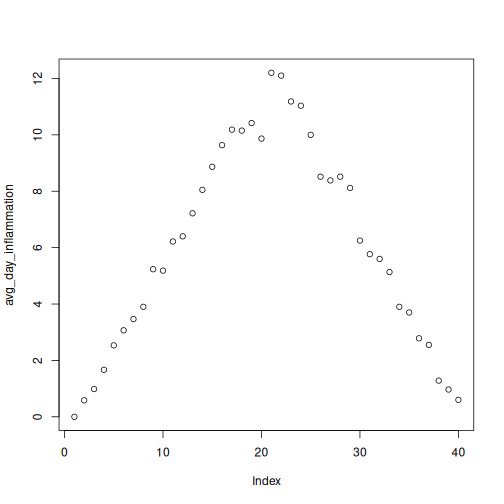## Making Choices

### Figure 7## Introduction to RStudio

### Figure 2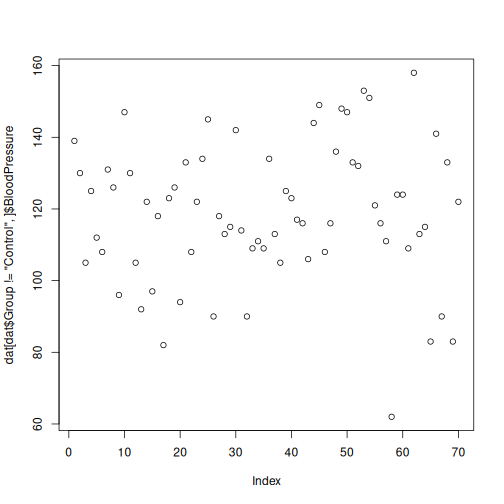2. In addition to dat$Group != 'Control', one could use dat$Group %in% c("Treatment1", "Treatment2").